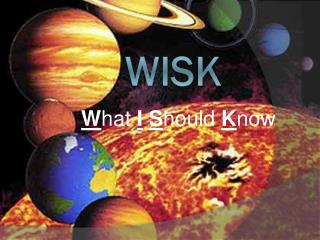Download PresentationWISK

# WISK - PowerPoint PPT PresentationDownload Presentation## WISK

- - - - - - - - - - - - - - - - - - - - - - - - - - - E N D - - - - - - - - - - - - - - - - - - - - - - - - - - -
##### Presentation Transcript

1. Explain the relationship between the Earth and the Sun’s gravity. • The Sun’s gravity pulls the Earth into orbit around it. • The Sun’s mass increases the pull of gravity and causes all planets to orbit the sun.

2. Explain the relationship between the Earth and the Moon’s gravity. • Both the Earth and the Moon have gravity. • The Earth’s gravity has a greater effect because of it’s mass. • The Earth’s gravity pulls the Moon into orbit around the Earth. • The Moon causes the tides of the Earth.

3. What is a tide and how does the motion of the Earth and Moon effect them? • A tide is the affect of the Moon’s gravity on the oceans of Earth. • As the Moon orbits the Earth, it’s gravity “pulls” the oceans towards it, causing a tidal bulge. • A tidal bulge is experienced on both sides of the Earth due to gravity and inertia.

4. Tidal Bulge

5. How does orbit effect the length of a year, month, and day? • A year is the amount of time it takes the Earth to orbit the Sun. • A month is the amount of time it takes the Moon to orbit the Earth. • A day is the amount of time it takes the Earth to rotate one time on it’s axis.

6. Orbit is caused by 2 forces. What are these forces and how do they work together to create an orbit? • Gravity and Inertia • Inertia is the force that keeps an object moving in a specific direction. • Gravity is the force that “pulls” an object towards it. • In space, an object will keep moving using only inertia, until it is attracted by the gravity of another object. • The combination of the two cause an object to orbit another.

7. What causes a solar eclipse? There will be 4 separate partial solar eclipses this year. None of which will be visible in North America

8. What causes a Lunar eclipse?

9. Moon Phases Identify the phases of the moon.

10. Explain the forces involved in the following picture? • Pulling force of soldiers. • Pulling force of helicopter. • Gravity on helicopter and soldiers. • Friction of flight line. • Air resistance.

11. How does the mass of an object affect it’s gravity? • The larger the mass, the more gravitational force it has. • A direct relationship between mass and gravitational force • You would way the most on Jupiter, because it has a greater mass than the other planets. • What problems would this cause astronauts?

12. What would life be without gravity?

13. Explain inertia. The force that keeps an object moving in the direction of the force.

14. What’s Next? Describe what causes the seasons. • Give your best detailed, possible explanation!

15. Describe what you see

16. Seasons are caused by. . . • A combination of the Earth’s orbit around the sun and the tilt of the Earth • Astronomy Connections: Earth in Motion